Technical Article

# AC-Equivalent Circuit Modelling

June 01, 2015 by Editorial Team

## The basic converters complete equivalent circuits can be studied here which include the basic AC modeling approach and the results for several converters. A simple circuit diagram is used for high-frequency converters. We will construct the non-linear model and convert it into a linear circuit that describes the small-signal performance of a circuit.

Discussions About Small-Signal AC-Equivalent Circuits of Several Common Converters

Beginner

#### Introduction

In some of the technical articles, the converter operation is studied in ideal conditions. An ideal condition is when there is no interruptions, disturbances and errors that cause the operation to deviate from its normal condition. These inevitable interruptions from normal conditions may be due to variations in the circuit parameters, such as voltages of source and load, switching time, and circuit components like inductors or capacitors. This behavior of the system is known as the dynamic system behavior, which needs to be rectified for proper output through a control mechanism. This requires an analysis and design of the controller by modelling approach which gives us the wide spectrum to analyze the various interruptions or issues.

Control system controls the parameters of the circuit by measuring the disturbances through open-loop or closed-loop system. Open-loop controller can minimize the anticipated disturbances by feed-forward path as shown. However, open-loop system alone can’t serve the need of the dynamic control for conversion. Here, closed-loop system can be used to measure the present behavior of the system and to take proper actions through feedback. The configuration for the control system is shown below in Fig.1.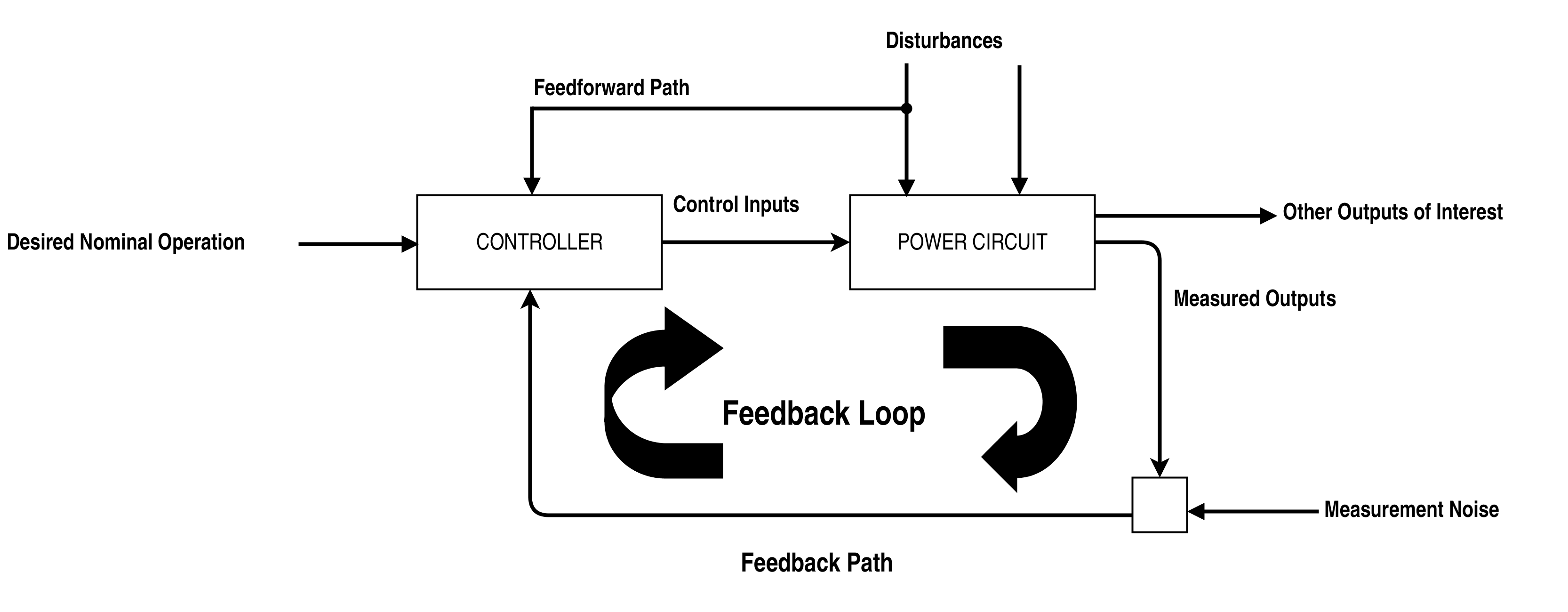Figure 1. General Configuration of Control System

There is a need to know the static as well as the dynamic behavior of the converter in designing the components for the required operating modes. Switching converters are a time-variant, non-linear and discrete system so the system must be made less sensitive to load or disturbances in the line.

The closed loop controller sets the output by noticing the deviations of the input and changing the parameter such as firing angle. Closed loop has an advantage that lets the controller also control the transients appearing during the switching of output. Feed-forward path alone can’t set the desired value of the output and gives the transients which are outside the allowed limits.

One of the solutions in maintaining the output voltage or current is to use a PI or PID controller. When it comes to steady-state errors, the PID controller is faster compared to the PI controller due to its derivative error/output inclusion.

In this technical article, the techniques to obtain suitable model for non-linear and linear converters are illustrated. Converter models will be made linear for continuous or discontinuous conduction mode for simplicity. Thus, special attention is intended for linear time invariant (LTI) models. Linear models are used for creating equivalent circuits.

#### The Basic AC Modelling Approach

There is a need to select the proper model for the different stages. For example, a closed loop controller is not a good choice for open loop performance of the circuit at a particular stage.  There are different approaches to design the model of a converter. Here, we can start our discussion with circuit average design of model which describes the average performance of converters for analysis, but It is not always the best method. Sometimes, circuit methods or averaging methods are not always appropriate and convenient for modeling controllers, so we will also see the state-space modeling approach.

Dynamic Models by Circuit Averaging

Simple circuit diagram is used for high-frequency converters. We will construct the non-linear model and convert it into a linear circuit that describes the small-signal performance of a circuit.

Switching operation of the power converter causes the pulse frequency for currents and voltages in the components. But it is difficult to consider these pulse frequencies of voltages and currents for stability purposes. For the dynamic analysis of the converter, it is required to take another method, then go for a detailed switching pattern behavior or pulsation in the pulse frequency, resulting in the dynamic averaging method.

In many of the power electronics applications, average values of current and voltage is of more value than the instantaneous values, provided that the value of harmonics and ripples are small enough for it to be ignored. Thus, we can take the average of a variable such as voltage or current using the circuit approach. The average at any time is taken over the interval T which is the shortest repeating switching interval related to the process of power circuit.

$$\overline{x(t)}= \frac{1}{T}\int_{ta-T}^{ta} x(t)dt$$

Average is taken over the length, T. Thus, $$\overline{x(t)}$$ is more flat as compared to x (t).

If x(t) has oscillations of particular frequency $$f_{C}=\frac{c}{T}$$. Then, these frequency components are nullified during the dynamic averaging.

These average variables also satisfy the fundamental equations i.e. KCL and KVL.

Thus, we can say

$$\overline{V_{R}(t)} = R\overline{I_{R}(t)}$$

$$\overline{V_{L}(t)} = L\frac{d}{dt}\overline{I_{L}(t)}$$

The sequence to apply the averaging and differentiation is interchangeable (i.e. any method can be applied first and then later other one).

Similarly for a capacitor, we have the following equations:

$$C\frac{d}{dt}\overline{V_{C}(t)}=\overline{I_{C}(t)}$$

With these types of fundamental equations we can create an average circuit model.

We have to change all of the instantaneous values with the average values without altering the LTI components. The linear parts of the circuits are not altered as they impose the same constraints on the normal as well as deviated variables. However, time-varying components and non-linear components are altered with the representation of an equivalent circuit that has some value of average voltage or average current. For instance, BJT switch is replaced with the small-signal model as shown below: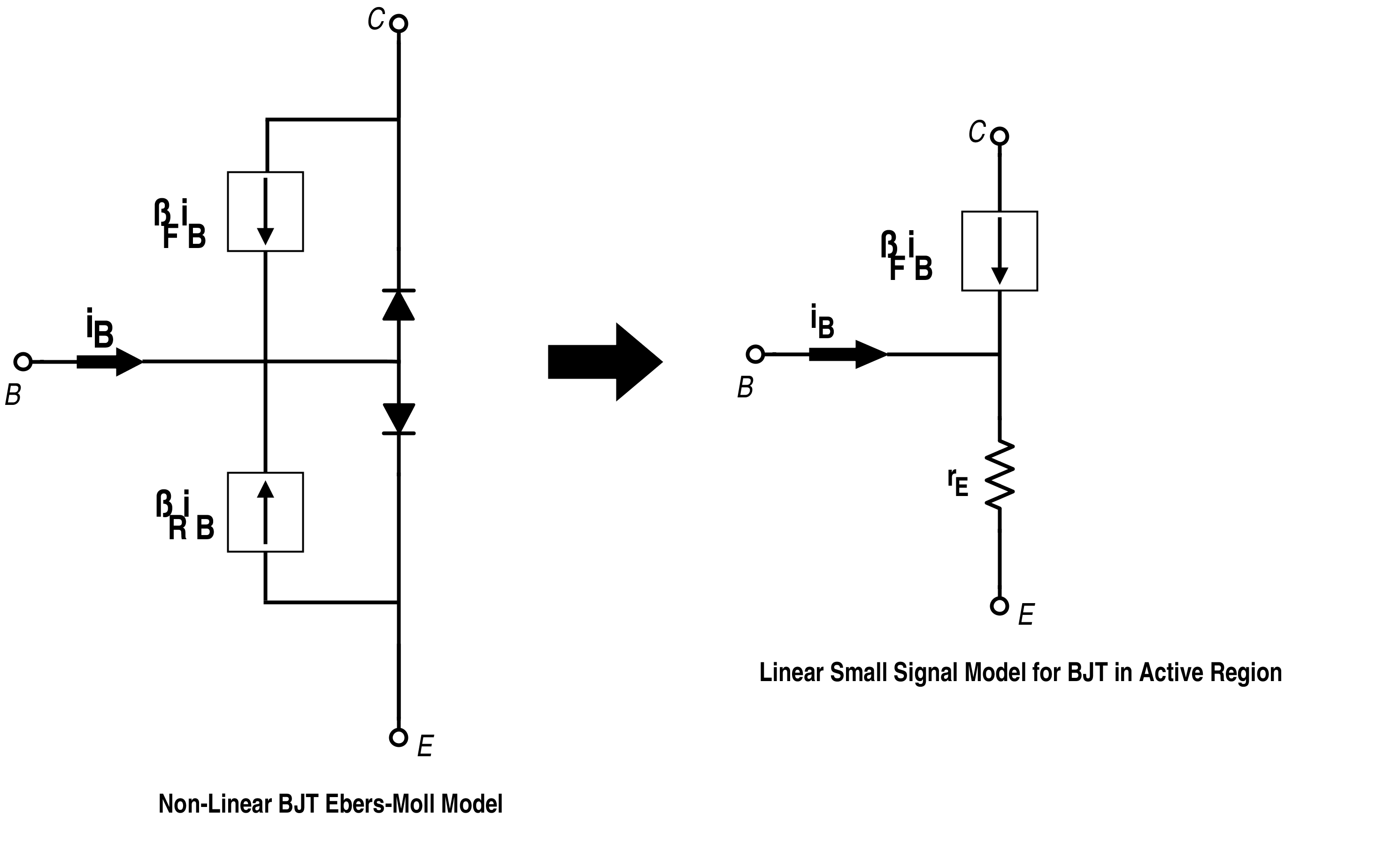Figure 2. Non-Linear Model and Small-Signal Model for BJT respectively

Linear models are also known as small-signal models, which simplify the judgment to analyze the non-linear circuit by making it approximately linear. We can also analyze the stability for normal operating condition with small deviations. Thus, the starting aim of the control designs is to stabilize the linear model than the non-linear model which can become cumbersome.

Consider a voltage source and a circuit represented by equivalent Norton circuit with the converter in middle as shown in Fig.3.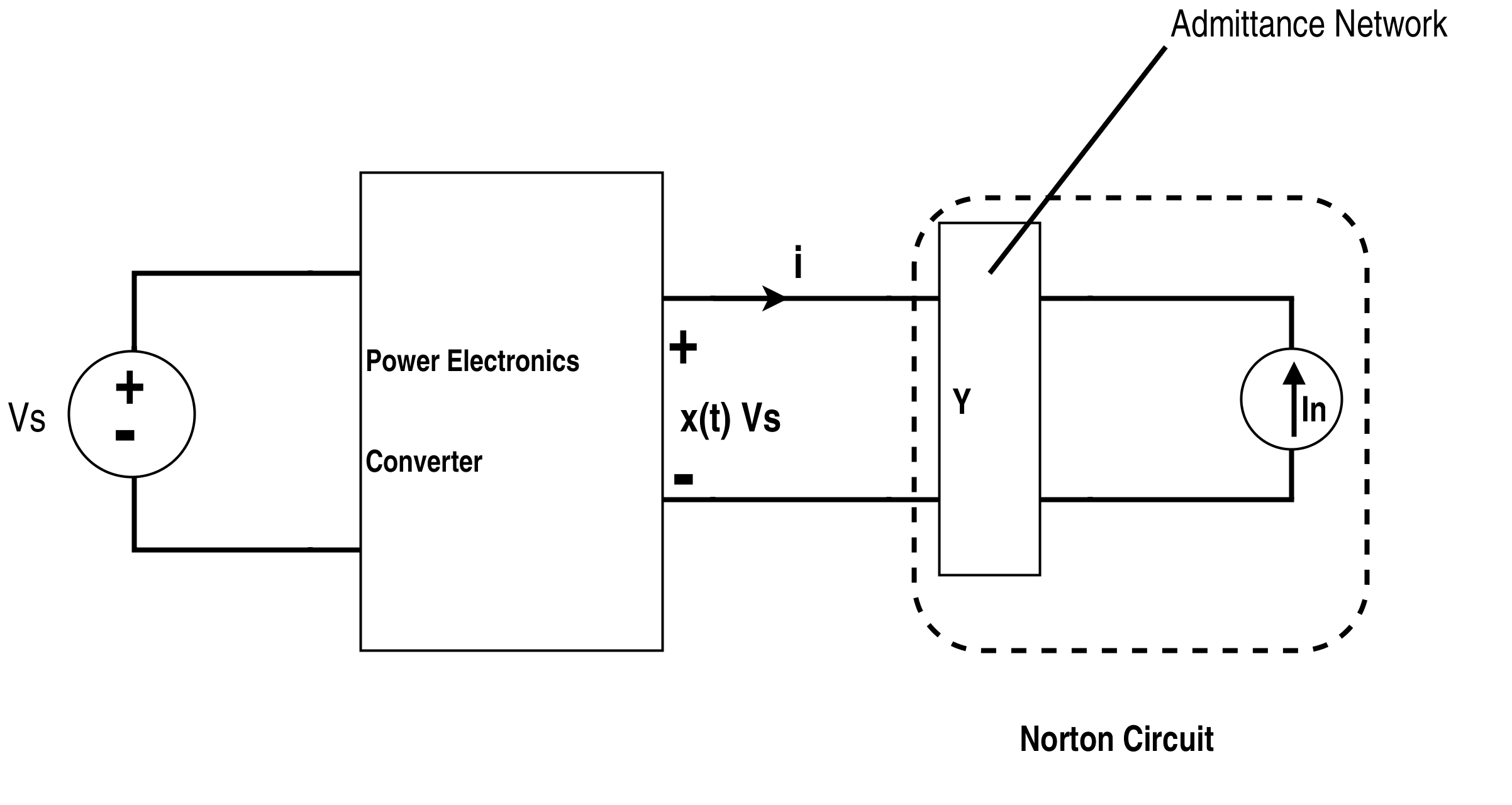Figure 3. General Switch Circuit

Here, x (t) is a switching function that modulates versus, the source voltage. This is dependent on the duty ratio of the switches. The value of q(t) set between finite values such as 1 and 0 for buck converter or 1, 0, -1 for the PWM inverter. The average circuit for this basic switched circuit is shown in Fig. 4.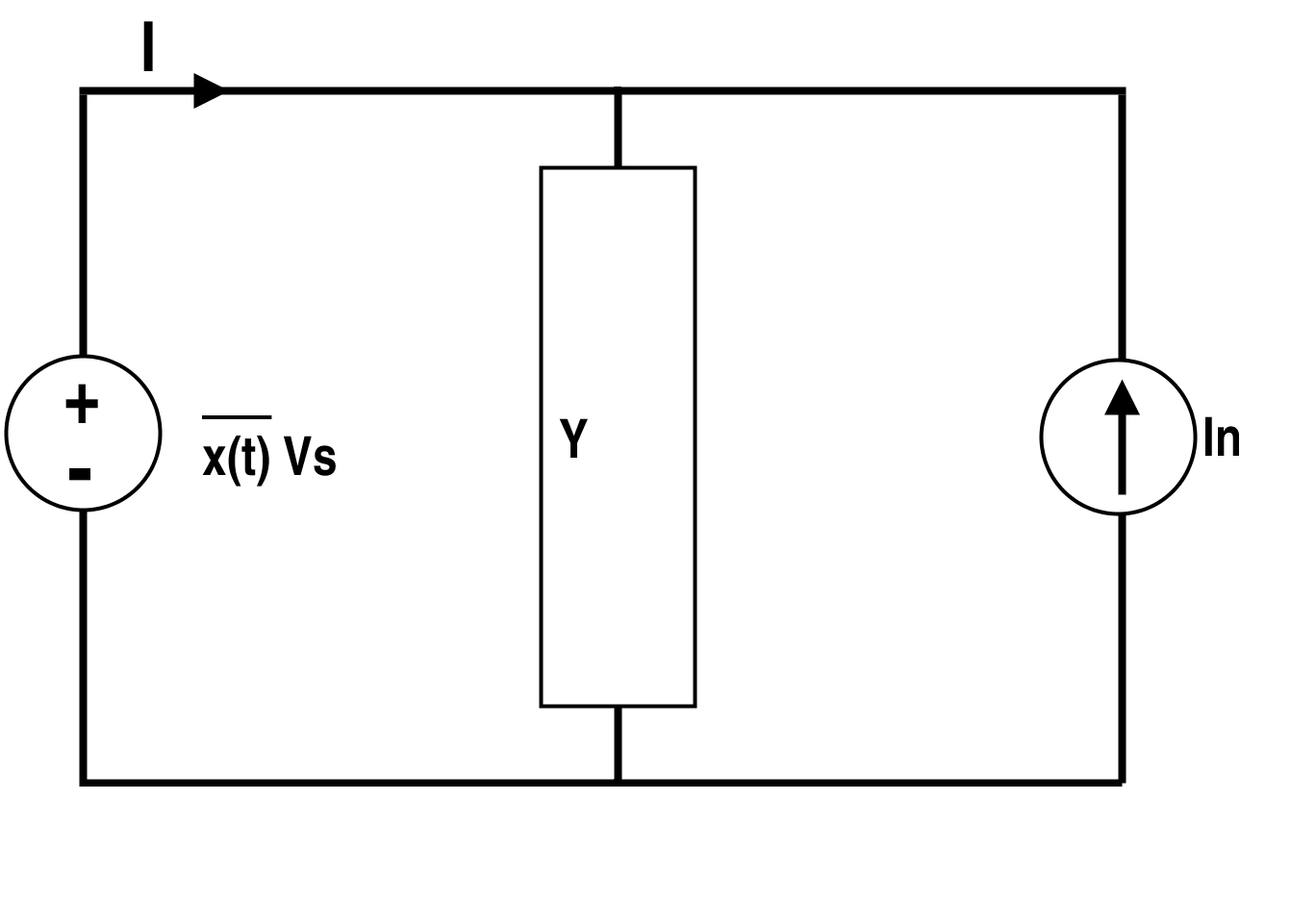Figure 4. Average Circuit for the Above Circuit

The function $$\overline{x(t)}$$ is an average switching function and also called continuous duty cycle ratio. $$\overline{x(t)}$$ is dependent on the control variables and average value of current i.e. $$\overline{I(t)}$$ entirely. This is generated with the help of circuits utilizing comparator, latch, clock, etc. The switching function is at the output of the latch. Latch is connected to the clock and the output of the comparator. Output of the controller, which is a modulated continuous average value wave of duty cycle, is connected to the comparator. Clock can be a sawtooth waveform that is applied to the positive terminal of the comparator.

Average value of voltage = $$\overline{x(t)} V_{S}$$.Thus,  $$\overline{x(t)}$$ vary inversely with the input voltage. Thus, disturbance in the output due to variation in the input voltage is restricted in the average circuit. The feedforward control for input voltage reduces the transient and steady state errors effect on the output.

$$\overline{x(t)}$$  is time varying and can even be negative. But, if x(t) is of constant period T without any deviation in switching frequency then $$\overline{x(t)}$$ is constant.

Replacement of switch with the linear small signal model gives us the variables which can control the performance of the model. Fig. 5 shows the standard circuit of a switch which can be replaced by the average circuit for continuous conduction in Fig.6. Average circuit can also be represented using ideal transformer as shown in Fig. 7.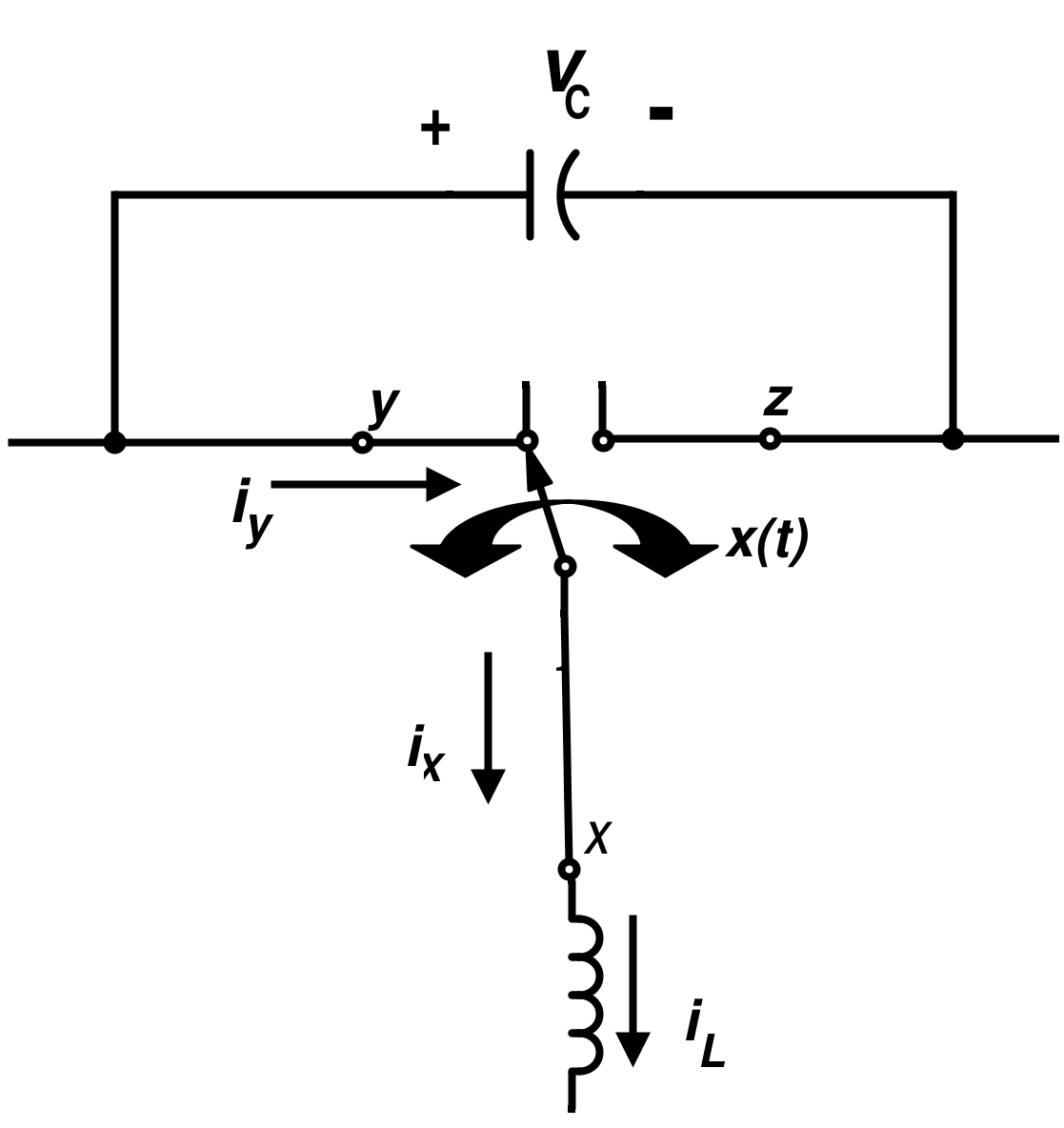Figure 5. Standard Switch Circuit

Assume that there are only small ripples, then load current and capacitor voltage can be well approximated by their average values. Also, it is assumed that the load voltage, source voltage and capacitor voltage do not vary considerably over an interval of length T.

If we assume iX(t) nearly constant due to small ripple approximation and slow variation of average value over the period T, then

For the interval t-T ≤ r ≤ T,

$$\overline{i_{Y}(t)}=\overline{x(t)i_{X}(t)}$$

Here the continuous duty ratio is $$\overline{x(t)}$$ = D (say), then

$$\overline{i_{Y}(t)}=D \overline{i_{X}(t)}$$

And $$\overline{v_{XZ}}=D\overline{v_{YZ}}$$

$$\overline{V_{L}(t)}=\frac{1}{T}\int_{ta-T}^{ta}V_{L}(t)dt=D\overline{V_{XZ}(t)} + {D}' \overline{V_{YX}(t)}$$ where, $${D}'=1-D$$

$$\Rightarrow L\frac{d}{dt}\overline{i_{X}(t)}=D\overline{V_{XZ}(t)}+{D}'\overline{V_{YX}(t)}$$

Similarly, for the capacitor (if the load R is connected on the xy side)
$$C\frac{d}{dt}(\overline{V_{C}(t)}) = -{D}'\overline{I(t)}-\frac{\overline{V(t)}}{R}$$

Now, the inductor volt balance condition and capacitor charge balance condition do not hold. But, it is true for nominal values. Moreover, these equations signify that there exist a separate voltage and current source to represent the deviations.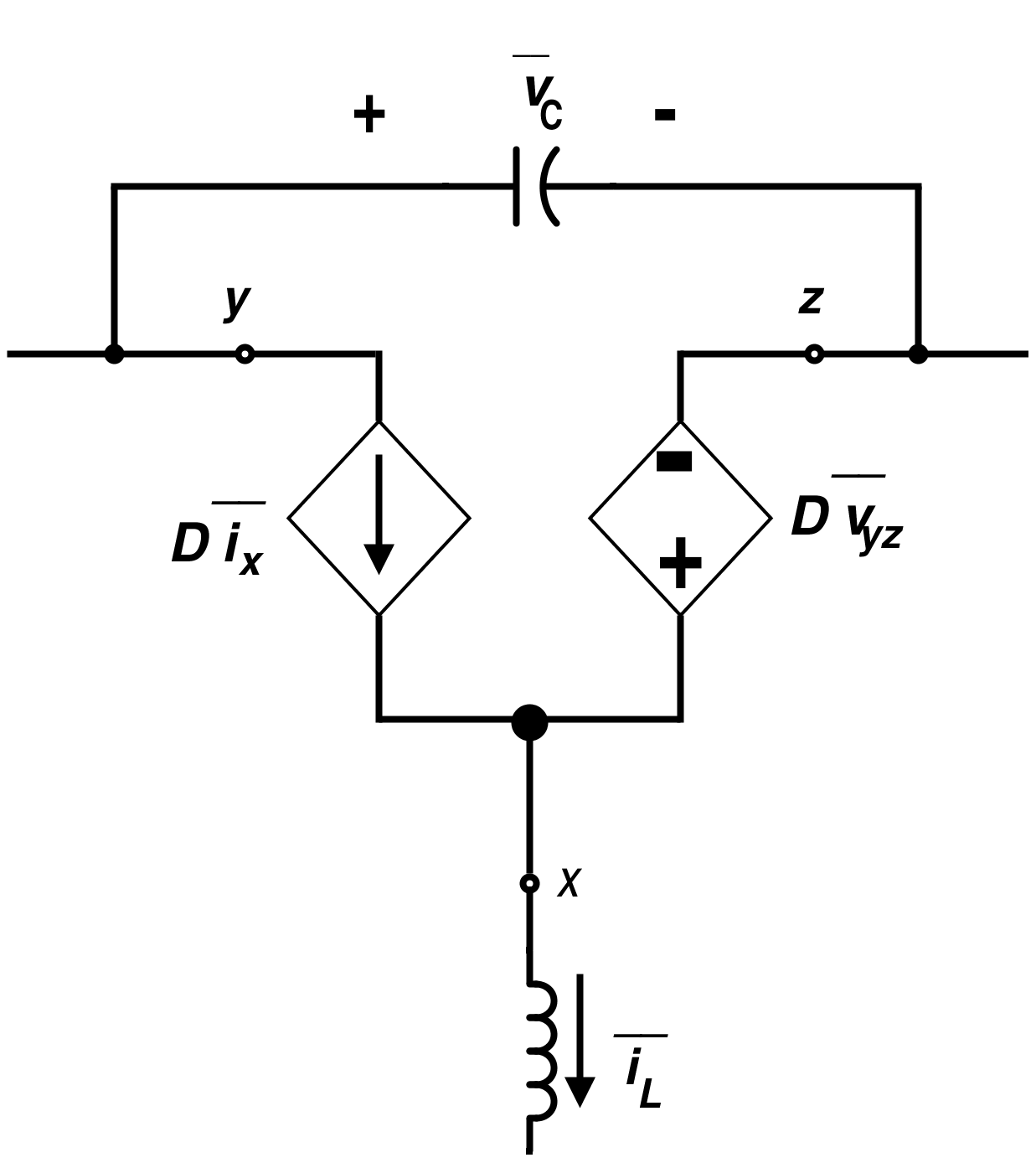Figure 6. Average Circuit for a Standard Switch Under Continuous Conduction Mode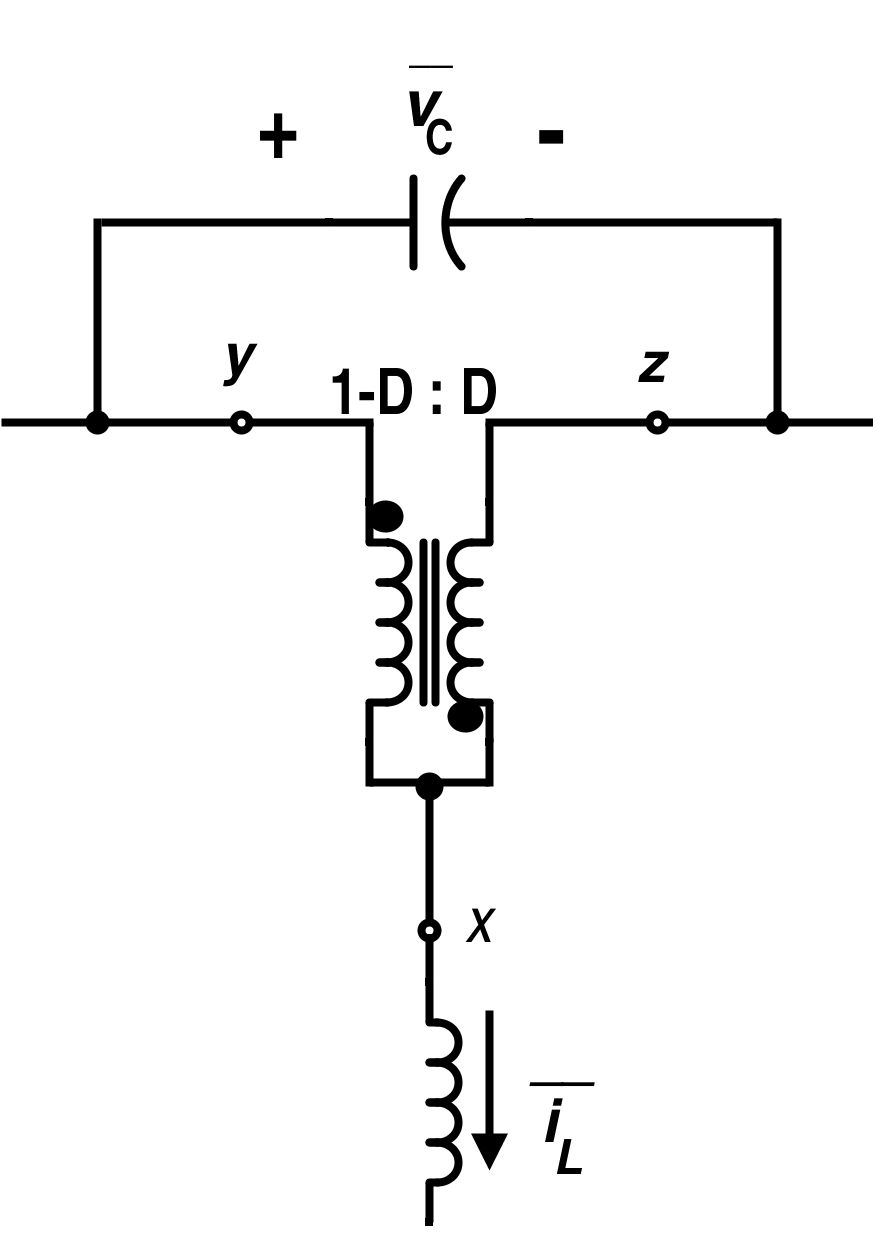Figure 7. Average Circuit for Standard Switch Using an Ideal Transformer

We can further simplify this circuit for linearization.

Consider that the circuit is initially in steady-state such that the average and instantaneous values are equal such as $$\overline{V_{S}(t)}=V_{n}(t)$$, where Vn(t) is the normal operating voltage. Now, if there is any small deviation from the normal condition. Then every voltage in the non-linear circuit is replaced with two sets of voltage or current to account for deviation. The system imposes the constraint on the original variable as well as deviation.

Due to small signal assumption, we have now,

On source side

$$|v_{S}|(\check t)|\ll|V_{n}|,$$

$$|d(\check{t})|\ll|D_{n}|,$$

$$|i_{s}(\check{t})|\ll I_{n}$$

$$|v(\check{t})|\ll |V|$$

$$|i(\check{t})|\ll|I|$$

Now, the linear circuit configuration will be as shown in Fig. 8. Let the deviation in continuous duty cycle is ď and Dn is the continuous duty cycle for the nominal value.

Thus,

$$D(t)=D_{n}(t)+d(\check{t})$$

$$\Rightarrow D(t)={D}'_{n}-d(\check{t})$$
Where

$$D(\check{t})=1-D(t) \; and \; {D}'_{n}=1-D_{n}$$

Also assume that the variation in input voltage is $$V_{S}(\check{t})$$

Hence,

$$V_{S}(t)=V_{n}(t)+v_{S}(\check{t})$$

Where Vn(t) is the normal operating voltage without any deviation.

On using these equations (including deviations) for inductor and capacitor, we will get the non-linear terms for the model. These equations need to be expanded using the Taylor series. If we retain the first order terms, we will get the linear model which also rule over the small deviations.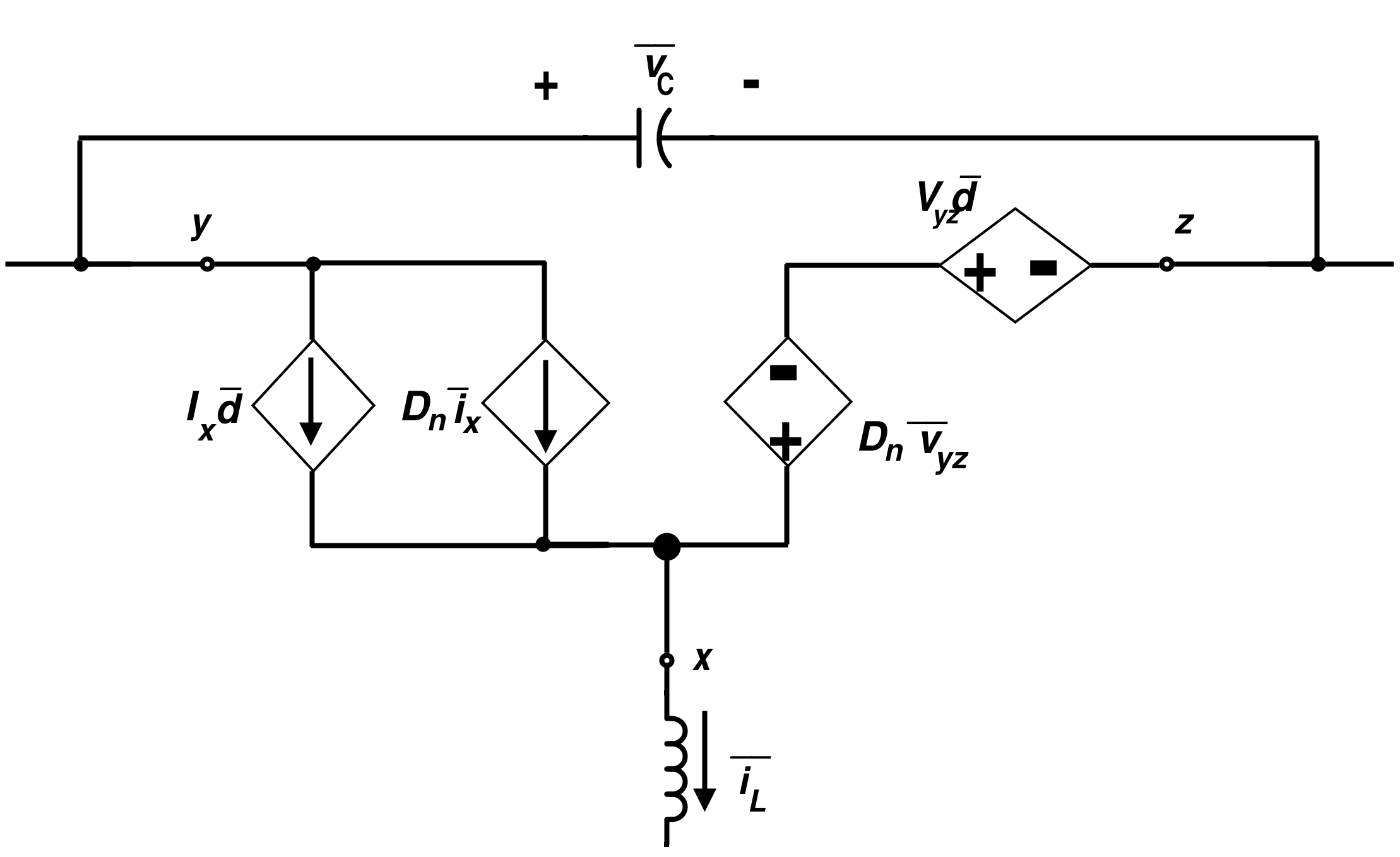Figure 8. Linear Average Model for the Standard Switch

Another representation using the ideal transformer for the linear model of the switch is shown in Fig. 9.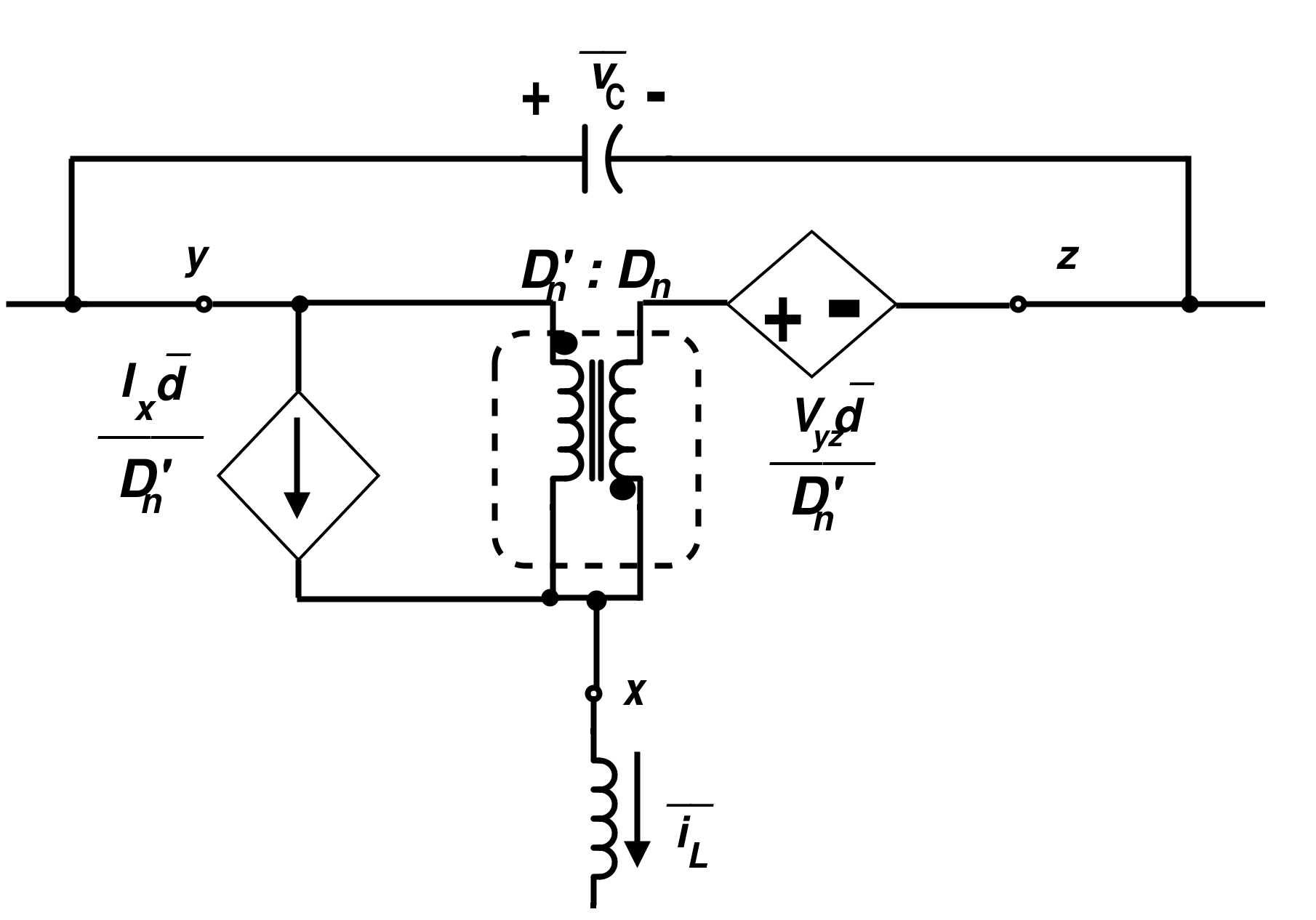Figure 9. Linear Model for Switch Using an Ideal Transformer

Shown in Fig. 10 is the configuration for the three different patterns for the switch connection. These linear models are valid for both AC and DC.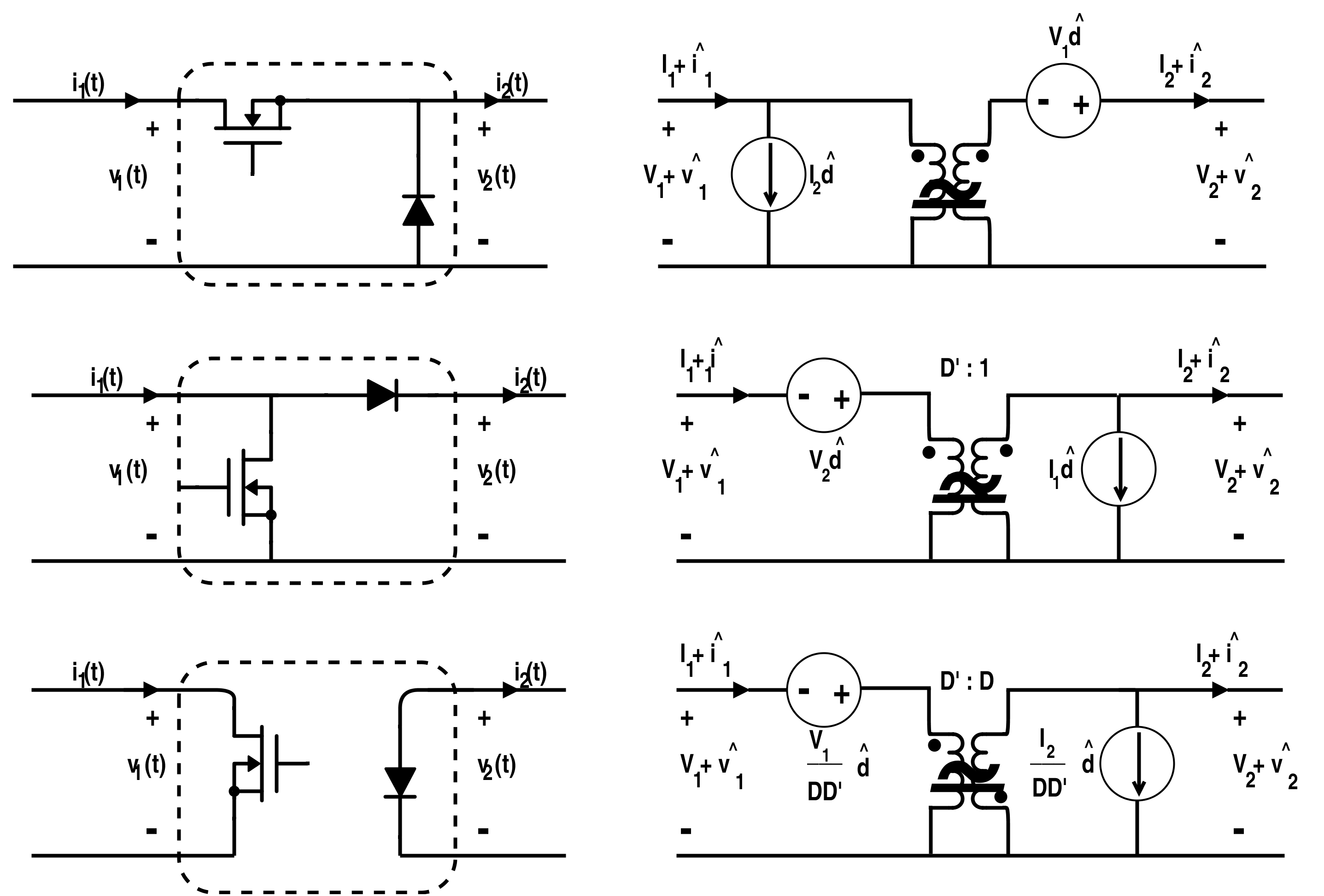Figure 10. Different Switch Configuration with their Linear-Model Conversion

The linear circuits shown below can even be designed for discontinuous conduction mode. In cases when the discontinuous conduction load R is high enough, the value of the inductor current reaches zero value. If the input voltage is constant over a switching period, the inductor current rises linearly until it decays to zero. When the current decays to zero, it can form a ringing circuit of RLC which is approximated by the linear segment. The different circuit patterns for the buck converter are shown below. Ringing circuit is shown in Fig. 11(b) which needed approximation for linearity.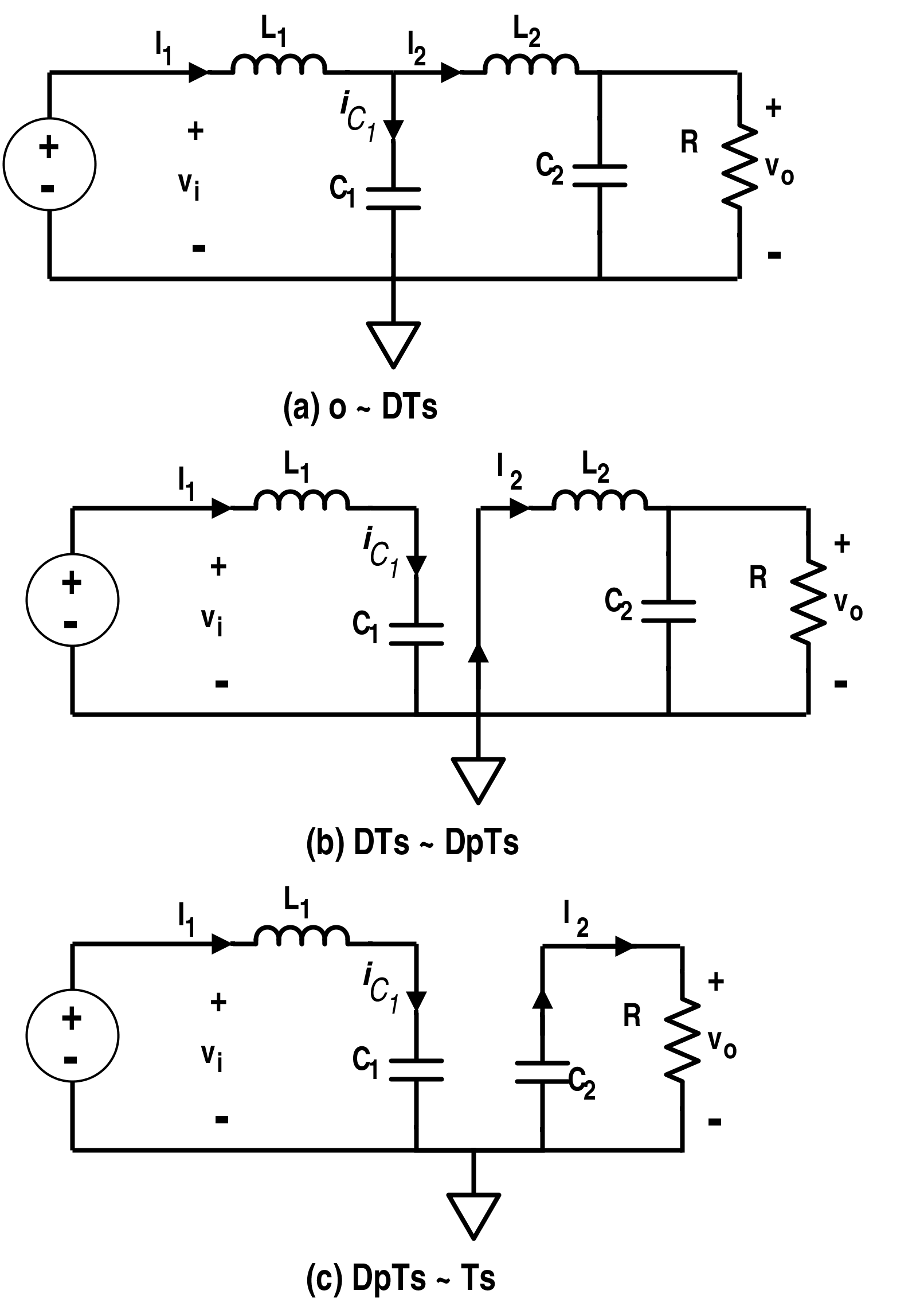Figure 11. Different Circuit Diagrams for Discontinuous Conduction Mode

#### Results for Several Basic Converters

Now, the linear circuits models and the switching converter circuits for the buck converter, boost converter, buck-boost converter and flyback converter are presented in Fig. 12 to Fig.19.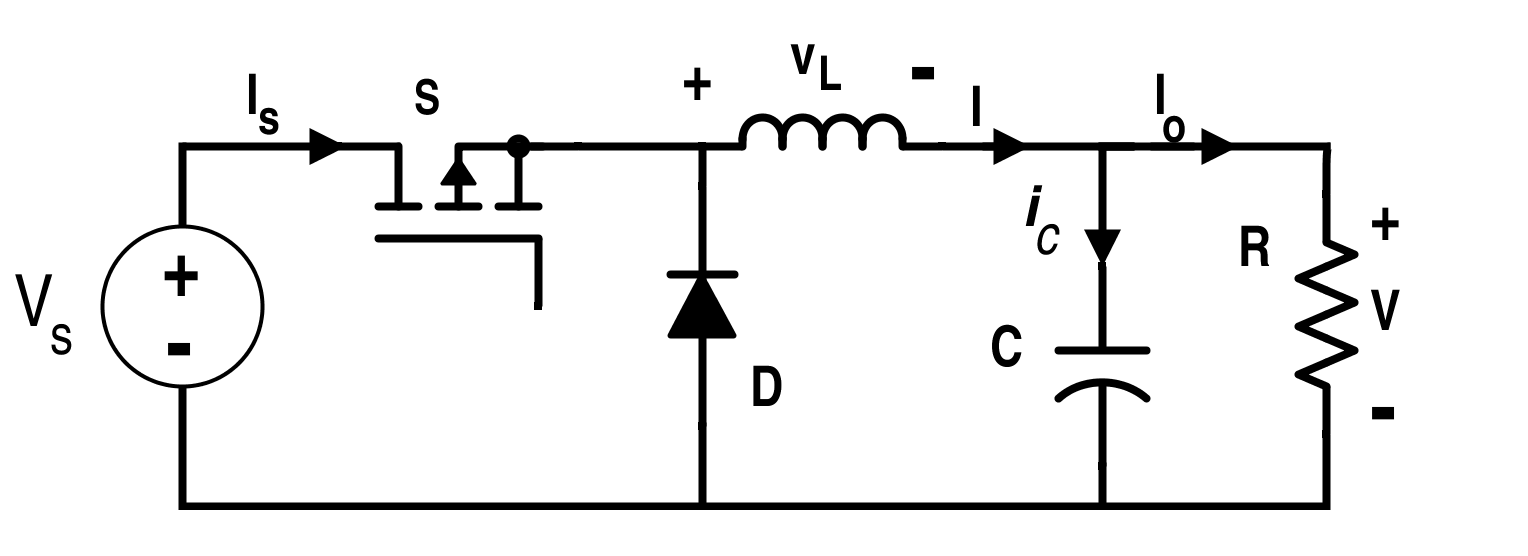Figure 12. Switching Converter Circuit for Buck Converter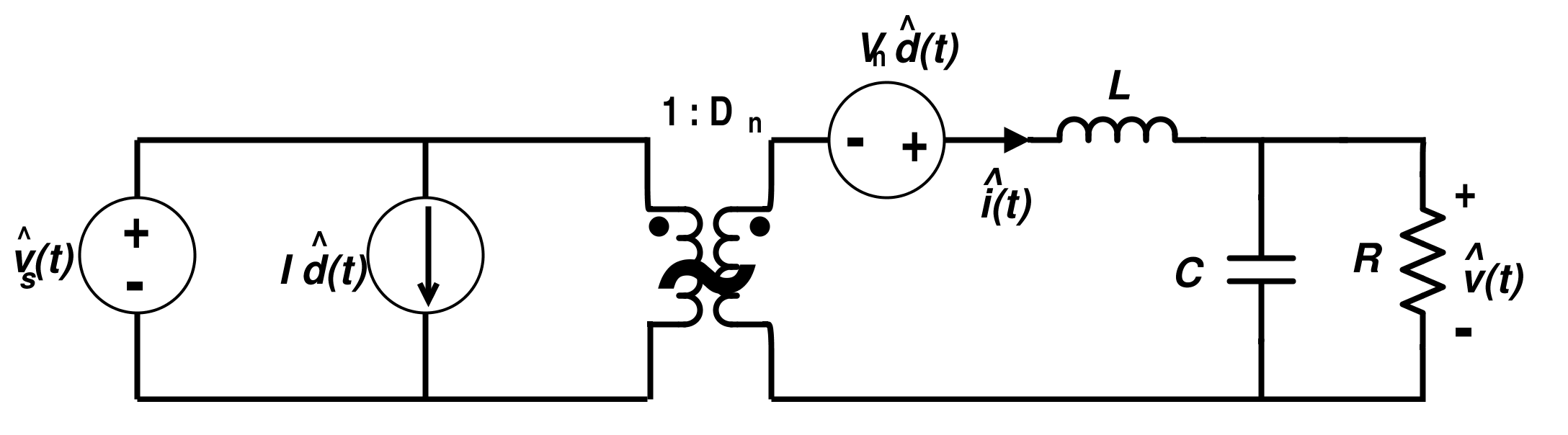Figure 13. Linear Circuit Model for Buck Converter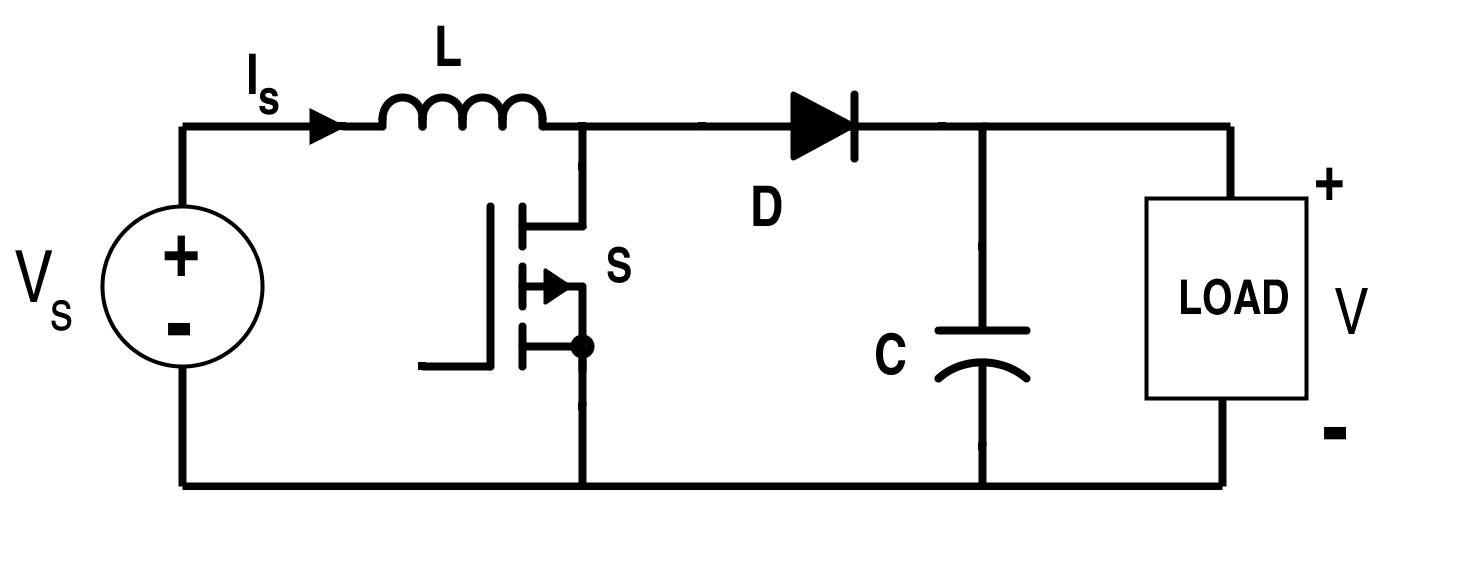Figure 14. Switching Converter Circuit for Boost Converter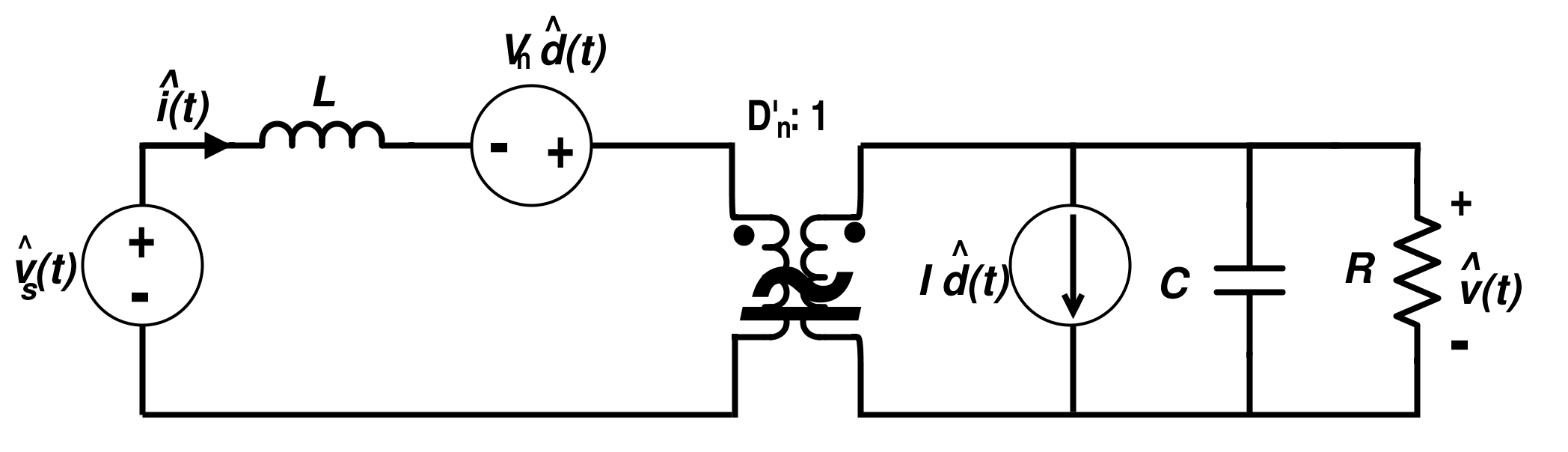Figure 15. Linear Circuit Model for Boost Converter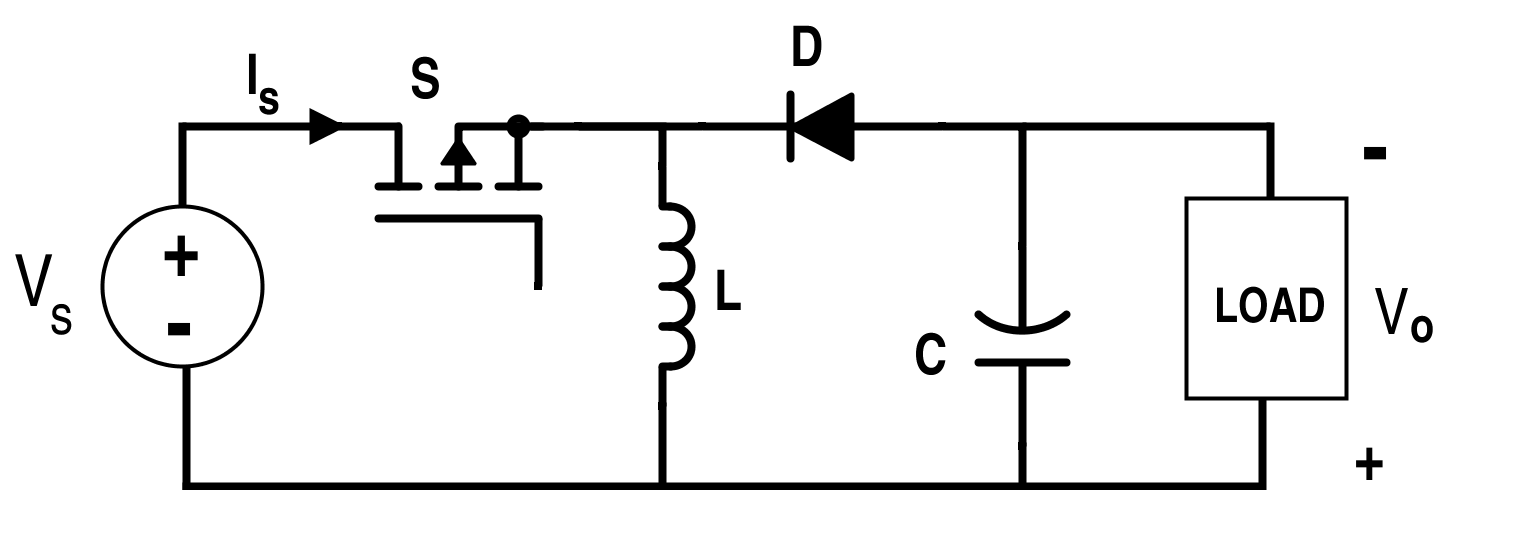Figure 16. Switching Converter Circuit for Buck-Boost Converter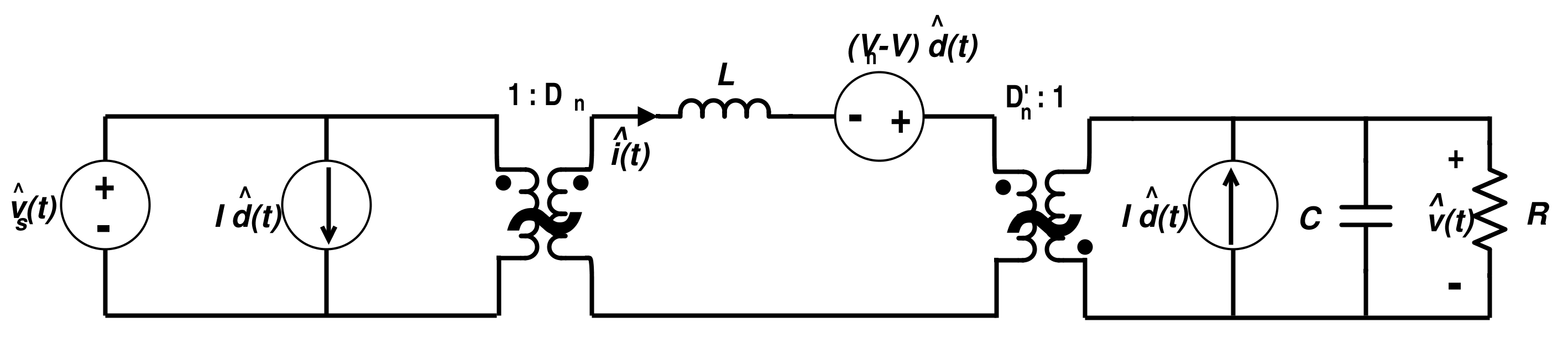Figure 17. Linear Circuit Model for Buck-Boost Converter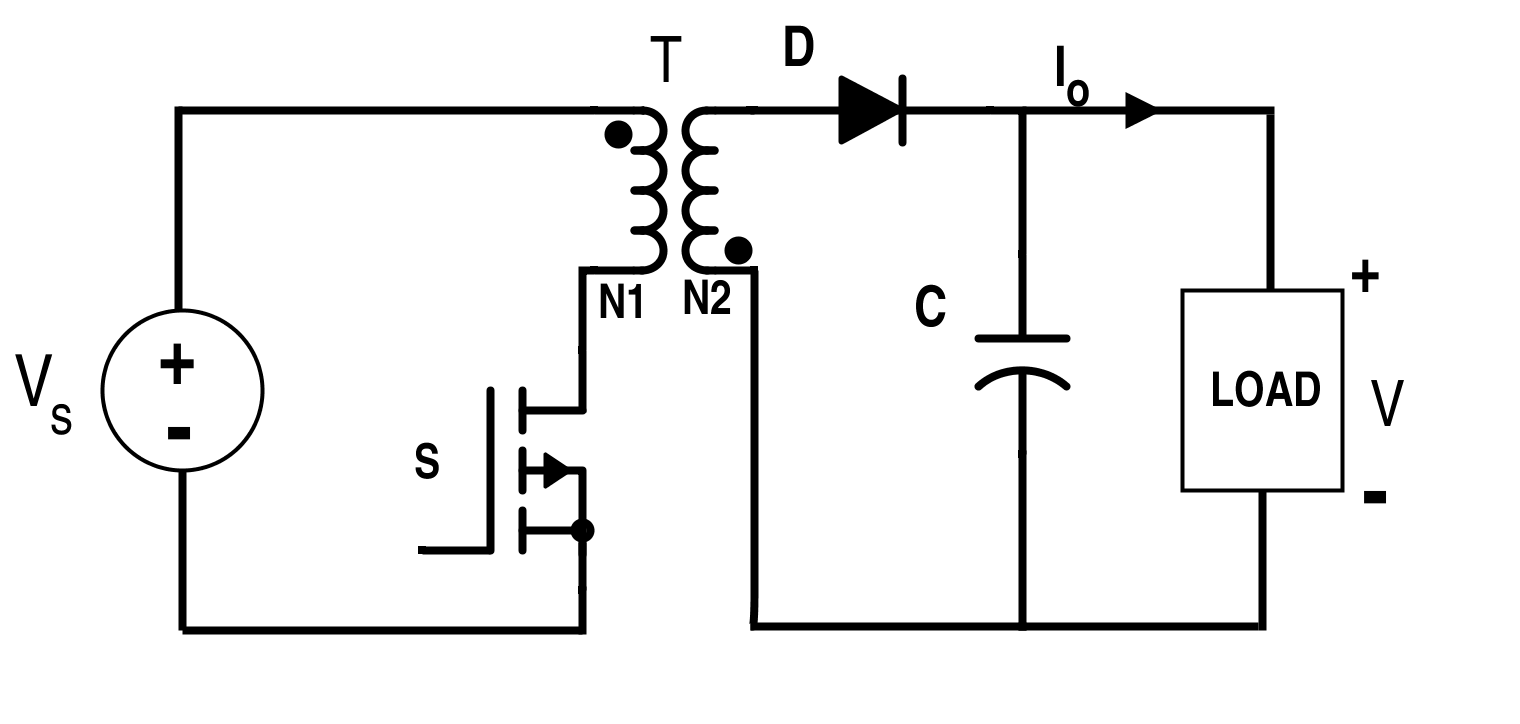Figure 18. Switching Circuit for Flyback Converter

Let the resistance of switch S during conduction period is  RON and $$n=\frac{N_{2}}{N_{1}}$$. Switch S and Diode D conducts alternatively.Figure 19. Linear Circuit Model for Flyback Converter